Speedometers, Thermometers, SWR Meters, and Smith Charts
Have you ever wondered what these four things have in common? No, of course not, me neither! But perhaps you have wondered why a Smith chart has such strange grid lines. Believe it or not, there is a logical progression from Speedometers to Smith charts that will make the Smith grid crystal clear.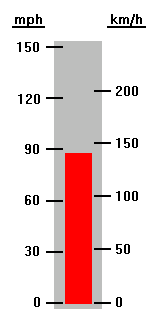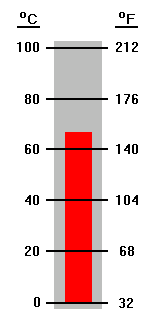These are stylized pictures of a speedometer and a thermometer. The speedometer indicates a speed that will get you a traffic ticket sooner or later. The thermometer might show the temperature of a cup of coffee. The speedometer is marked in both miles per hour and kilometers per hour. The markings are not aligned, allowing nice round numbers to be used on both scales. On the thermometer the markings are aligned but the Fahrenheit numbers obviously have been forced to match the Celsius scale. It doesn't really matter how the scales are marked. For both speed and temperature only one quantity is being measured, but the measurement is then shown with two different scales.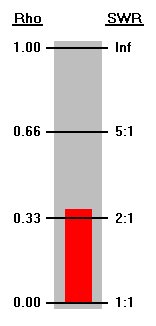This is an SWR meter. These meters don't actually measure the Standing Wave Ratio. They measure the magnitude of the reflection coefficient (rho) as compared to a given reference, usually 50 ohms. The scale may be marked in SWR units, but it is important to remember that the "real" (but perhaps not shown) scale is the reflection coefficient magnitude. The SWR meter is only concerned with the magnitude of the reflection coefficient, but rho is a complex quantity having both a magnitude and angle. If rho were to be measured at various points along a transmission line, the magnitude would be fairly constant but the angle would change depending on the electrical length of the line at the point of measurement.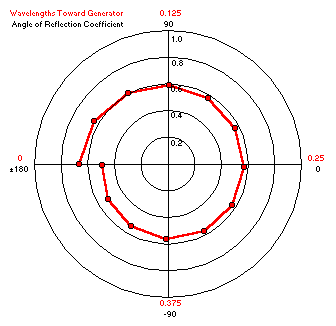This plot shows both rho magnitude and rho angle at points along a ½ wavelength section of RG-174 at 3.75 MHz. The line is terminated with a load of 10+j0 ohms. Calculations have been made every 15 electrical degrees working clockwise toward the generator end of the line. Rho magnitude is indicated by the distance out from the center of the chart and rho angle is measured from the 3 o'clock position.

Because RG-174 is a lossy line the plot is in the shape of a spiral rather than a circle. The reflection coefficient magnitude drops noticeably as the distance from the load is increased. Rho magnitude at the load is 0.667 (SWR 5:1) while after ½ wavelength it has dropped to 0.494 (SWR 2.95:1). The rho angle starts at (approximately) 180° and sweeps through an entire circle to end up back at (approximately) 180°.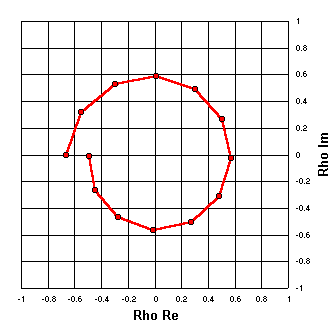The complex quantity rho can be expressed as real and imaginary components instead of as magnitude and angle. The scale markings on the plot at left indicate this, but notice that the shape of the spiral is exactly the same as with the previous plot. This is analogous to the speedometer and thermometer examples, where the same quantity may be shown with different scales.

Speed and temperature are of course scalar quantities that can be expressed with a single number. Rho is a vector quantity that requires two numbers in order to be completely defined. Trying to put both sets of scales on the same chart would be confusing, but the idea is the same.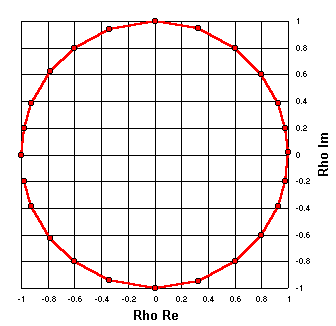R = 0

Instead of plotting reflection coefficients as would be found along a transmission line we can plot the reflection coefficients that correspond to arbitrary impedance values. For any arbitrary complex impedance Z (R±jX) the reflection coefficient may be calculated as

Rho = (Z - Zref) / (Z + Zref)

In this plot all the arbitrary Z values have a resistance of R = 0 ohms. The reactance values are X = 0, 5, 10, 17, 25, 35, 50, 70, 100, 150, 250, 500, 5000, -500, -250, ... , -10, and -5. (That is, Z = 0+j0, 0+j5, 0+j10, etc.) A reference impedance (Zref) of exactly 50+j0 ohms has been used for this chart and for all other charts on this page.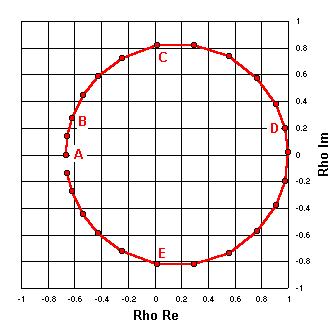R = 10

Now this process will be repeated, but this time the R component of the arbitrary Z points will be 10 ohms. The following table shows a few of the calculations.

Label     Arbitrary Z     Rho Re     Rho Im
A 10 +j 0 -0.667 0
B 10 +j 10 -0.622 +0.270
C 10 +j 50 +0.016 +0.820
D 10 +j 500 +0.976 +0.197
E 10 -j 50 +0.016 -0.820
Rho Zref = 50 +j 0R = 50

And again, but with R equal to 50 ohms. (Z = 50+j0, 50+j5, 50+j10, etc.)X = 0

Now let's plot the real and imaginary components of the reflection coefficients from a set of arbitrary Z values with the X component held constant. In this case R = 0, 10, 25, 50, 100, 250, 500, 5000 and X is held constant at 0.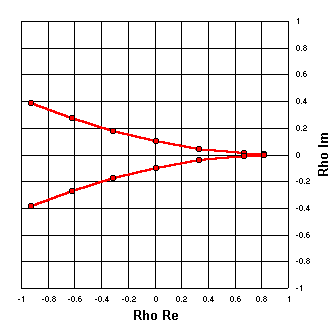X = ±10

You get the idea, so now let's show two sets of plots. The top arc is for R = 0, 10, 25, 50, 100, 250, 500 with X = 10, and the bottom arc is for the same set of R values but with X = -10.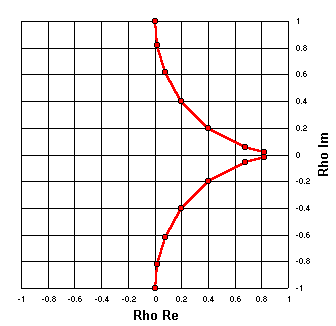X = ±50

One last time. Same set of R values as in the previous plot, with X = 50 and X = -50.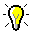Here are the previous six plots superimposed, with labels to indicate which component of the arbitrary Z values was held constant.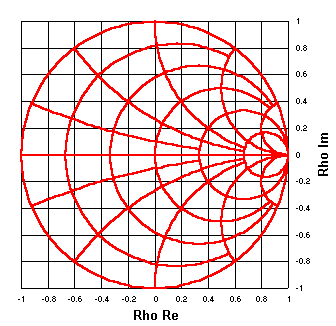Now we've added a few more points to each of the previous plots and removed the individual point markers in order to smooth the curves. We've also added plots for R held constant at 25, 100, and 250 ohms with X varying, and for X held constant at ±25, ±100, and ±250 ohms with R varying.Now the Rho Re and Rho Im grid lines and labels have been hidden. The heavy red plot lines have been changed to light black "grid" lines and labels have been added to show the constant R or X values which were used to create each line.

Note that this chart, just like the SWR meter, is still based on reflection coefficients. But now instead of the chart scales showing rho magnitude and angle, or rho real and imaginary components, they show impedance (R and X) values for a 50 ohm reference base. This is exactly like adding a third scale to the thermometer, perhaps degrees Kelvin, and then erasing the Celsius and Fahrenheit scales. The instrument would still measure temperature and you would still call it a "thermometer."

The chart at left could be called a "Reflection Coefficient Chart with Impedance Scale Markings," but most people choose to honor its inventor instead and call it a Smith Chart.(Hover mouse over any red marker.) (May not work with some browsers.) Remember the example of the RG-174 transmission line with calculations made every 15 electrical degrees? The same plot is shown at left, but with scale markings in terms of impedance values at points along the line rather than reflection coefficient values. Notice that the shape of the spiral is exactly the same. If you don't believe me just click one of the buttons.       Hover your mouse pointer over any red marker to see the exact values for that point, displayed either on the status line or in a pop-up box depending on your browser. (May not work with some browsers.) (How accurate are these charts?) And just for historical perspective, click here to see the same points plotted on a chart similar to the "precursor" Smith chart, circa 1931.

Home